# Numerical study of the chaotic flow in three-dimensional open geometry and its effect on the both fluid mixing and heat performances

Numerical study of the chaotic flow in three-dimensional open geometry and its effect on the both fluid mixing and heat performances

Ahmed L. BoukhalkhalYahia Lasbet Mohammed Makhlouf Khaled Loubar

Department of Mechanical Engineering, Djillali Liabes University, 22000 Sidi Bel Abbes, Algeria

LDMM Laboratory, Ziane Achour University of Djelfa, 17000 Djelfa, Algeria

GEPEA, UMR 6144, Ecole des Mines de Nantes, France.

Corresponding Author Email:
lamine_aa@yahoo.fr
Page:
1-10
|
DOI:
https://doi.org/ 10.18280/ijht.350101
|
Accepted:
|
Published:
31 March 2017
| Citation

OPEN ACCESS

Abstract:

In this study, we investigate the chaotic behavior of fluid particles in three-dimensional open geometries and their effects on the amelioration of miscible fluids mixing, thermal homogenization and thermal performances by using a computational fluid dynamics (CFD) method. Three geometry configurations (straight channel, serpentine–2D channel and chaotic geometry channel serpentine–3D) are considered. The dispersion phenomena are characterized through the presentation of the Poincaré sections and the mixing quality is quantified by calculating the mixing degree in cross section for several Reynolds numbers ranging from 5 to 200. This study reveals that height capacities in terms of mixing and heat performances are obtained by the chaotic geometry where the level mixing is superior to 0.95 for Reynolds equal to 200. In addition, the heat transfer in the S-3D is highly improved where the mean Nusselt number is 13 times bigger than that calculated in the straight channel.

Keywords:

Chaotic Advection, Mixing Degree, Nusselt Number, PoincarÉ Sections, Serpentine Channel

1. Introduction

The perfect mixing quality and a good thermal homogenization are required in many engineering applications such as heat exchanger, fluids mixing, oil and biotechnology industries…etc. The systems utilized to this end can be classified according to the flow regime that takes place in these mixers: turbulent flow, or chaotic laminar flow.

In turbulent flow field, the kinematic trajectories are three-dimensional and random, which leads to the high stirring rate and creates very thin structures. However, the turbulent flow has many disadvantages such as large energy dissipation rate in the flow and the alteration of the molecules composed of long molecular structures .

In the chaotic flow, there is a great sensitivity to initial conditions, leading to rapidly diverging trajectories for two originally neighboring particles which give chaotic and erratic behavior trajectories . In addition, the principle features of such flows are the existing of the stretching and folding processes. All of these phenomena are responsible for the destruction of the interface between the fluids and consequently the fluid mixing is improved without energy expenditure and dissipated energy compared to that dissipated in turbulent flows .

The advantages offered by a chaotic geometry used as a mixer and/or heat exchanger have been established in previous studies [4–8] when compared with a straight pipe or helically coiled pipe. Habchi et al.  studied the dispersion process (liquid / liquid), related to the mixing of immiscible fluids, in two types of geometries: helically coiled pipe and twisted pipe (chaotic geometry). Experiments showed the effect of chaotic advection on droplet breakup and provided smaller and more homogeneously dispersed droplets.

Zhou et al.  optimized the effect of the lateral structure of passive mixer to enhance the mixing efficiency. Their numerical results showed that the new geometry giving a better mixing degree than the conventional mixer for different Reynolds number. In an acceptable number of periods of serpentine–2D geometry, numerical studies have proved that existing of both chaotic advection and rigid particles enhanced remarkably the mixing quality . The mixing of two fluids in a planar serpentine convergent–divergent mini–channel has been studied numerically . This geometry led to a rapid chaotic mixing and the results revealed that the stretching and folding of interfaces was hence effectively enhanced. Habchi et al.  used longitudinal vortex generator mounted interior a circular channel is studied in which the array is rotated periodically by an angle of 900. The chaotic flows improved inside a circular duct the mixing efficiency by the stretching and folding phenomena. Recently, Karami et al.  studied numerically the effect of the geometrical perturbation and the flow pulsation in curved ducts under laminar regime flow. The results showed that the formation of homoclinic and heteroclinic connections due to the pulsatile flow enhanced the mixing.

Most of studied characterized a new type of geometry which can be a promoted generator of chaotic flows. Robin et al.  introduced and tested experimentally a chaotic three–dimensional serpentine microchannel as a means to passively enhance fluid mixing. Experiments using phenolphthalein and sodium hydroxide solutions demonstrated the ability of flow in this channel to mix faster and more uniformly than the square-wave channel and a straight channel for Reynolds numbers ranging from 6 to 70. Hwang et al.  investigated numerically the mixing of two miscible fluids in three different microchannel geometries without neglecting the molecular diffusion (1D straight channel, 2D square–wave channel and 3D serpentine channel) with an initial vertical injection position of the dividing surface at the entrance. The 3D serpentine channel is similar to the C–shaped microchannel geometry proposed by .

In the current study, we will examine, firstly, the local behavior of the velocity field such as pathlines, the particles dispersion and Poincare section. Secondly, the performances of the selected geometries will be checked in terms of the fluids mixing, temperature homogenization (thermal mixing) and parietal heat exchange.

It should be noted that in the previous works  and , the authors outlined the mixing into microchannels without including the effect of the injection mode. However, in the present study, the mixing is measured in minichannels for two injection modes: horizontal and vertical. In addition, contrary to the previous work and in order to highlight the chaotic effects, the molecular diffusion is not taken into a consideration.

Such design tested in this study, S–3D geometry, has already showed important thermal performances in heat exchanger PEMFC cooling [17-19]. It improved greatly current heat exchanger performances for PEMFC cooling applications. In this geometry, the convective heat transfer coefficient, Nusselt number, is about six times than that of the straight channel.

## 1.jpg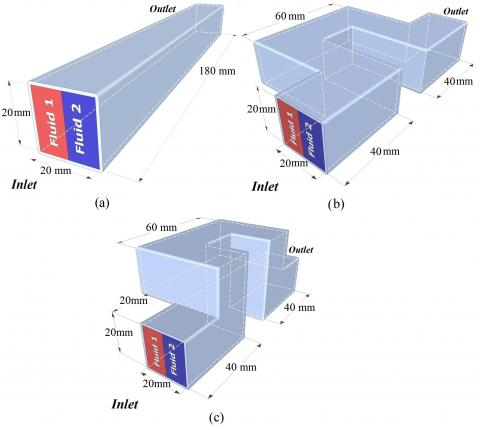Figure 1. Geometries considered: a) Straight channel (S-C), b) Serpentine-2D, c) Chaotic geometry (serpentine-3D)

2. Description of Geometries and Numerical Method

2.1 Geometries description

Three geometries are considered, straight channel (S–C), serpentine–2D and chaotic channel "serpentine–3D". Figure 1 presents the basic element of all geometries. To make up a complete channel, each period is repeated in space. For all geometries, the channel cross-section is square with an aspect ratio of 1 (20 mm × 20 mm). The hydraulic diameter Dh is 20 mm. The unfolded length of one period is equal to 180 mm.

Figure 1a shows the classical geometry straight channel. The geometry called (S–2D) is a serpentine geometry shown in Figure 1b. It is formed by a succession of 90° sharp bends in the same plane. Geometry Serpentine–3D (Figure 1c) initially introduced by Robin et al. , generates spatially chaotic flows as shown by the work of Beebe et al. . It is formed by two geometric perturbations, each perturbation formed by a succession of three 90° sharp bends in different plane.

2.2 Numerical method

The conservation of mass and Navier–Stokes equations are numerically solved using the commercial CFD code ANSYS Fluent. In this study, the fluid is considered incompressible and the flow is steady and laminar. These simulations are made ​​with the volume of fluid (VOF) solver with an implicit scheme implemented in this code. The SIMPLE scheme is used to achieve the pressure-velocity coupling, and in spatial discretization, a Second-Order upwind scheme was selected in momentum, energy and volume fraction, a PRESTO was applied for the discretization of pressure. It is considered that convergence is achieved when the residues are less than 10-4 for the conservation equation and 10-6 for the volume fractions and energy equation. The imposed boundary conditions are detailed in Table 1. The simulations are limited for each geometry to two period.

Table 1. The imposed boundary conditions.

 Boundary Boundary conditions Case 1 Inlet Uniform axial velocity with a volume fraction equal to 0 for fluid1 and equal to 1 for fluid2. Outlet The outflow condition. Wall No-slip Case 2 Inlet Uniform axial velocity with a temperature equal to 300 k for fluid1 and equal to 320 K for fluid2. Outlet The outflow condition. Wall No-slip with adiabatic walls. Case 3 Inlet Uniform axial velocity with temperature equal to 300 k. Outlet The outflow condition. Wall No-slip with a uniform heat flux condition

## 2.jpg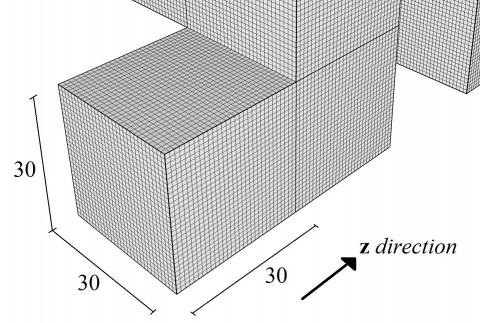Figure 2. Example of structured grid system

2.3 Grid independence

A parametric study was performed to identify the grid mesh that assures the capture of volume fraction and velocity gradients near the walls. The hexahedral grids for the full model are used (Figure 2). Four different grids mesh, namely: 30×30×30, 35×35×35, 40×40×40, and 45×45×45 were tested to validate the appropriate grid mesh. The simulation tests on the velocity profile and the volume fraction are shown in Figure 3. A spatial resolution of 40 × 40 meshes in the cross section (in the x and y directions) and 40 meshes in 20 mm in the z direction was found to be fully satisfactory.

2.4 Characterization of the mixing and parietal heat exchange

Mixing performances in the considered geometries are assessed using the degree of mixing as defined in previous works [18, 21, 22] as following:

$D\,=\,1-\frac{\sqrt{\frac{1}{N}{{\sum\limits_{1}^{N}{\left( {{X}_{i}}-\overline{X} \right)}}^{2}}}}{{{\sigma }_{0}}}$                (1)

where:

$\overline{X}=\,\text{ }\frac{1}{N}\sum\limits_{1}^{N}{\left( {{X}_{i}} \right)}$              (2)

N is the number of nodes inside the cross section.

For the fluid mixing, Xi is the volume fraction of fluid2 at the node i, $\overline{X}$ is the arithmetic average volume fraction in cross section and it is equal to 0.5. For the thermal mixing, Xi is the static temperature at the node i and $\overline{X}$ is the mean temperature at the cross sectionand where it is equal to 310K.  ${{\sigma }_{0}}$ is the standard deviation at the inlet section of the geometry as defined as follow:

${{\sigma }_{0}}=\sqrt{\frac{1}{N}{{\sum\limits_{1}^{N}{\left( {{X}_{i}}-\overline{X} \right)}}^{2}}}$            (3)

As defined, the degree of mixing varies from 0 to 1; thus, for a fully mixed channel, D = 1.

In order to characterize the heat transfer performances in the channels, we follow the evolution of the local Nusselt number Nu along the curvilinear coordinate S such as defined as bellow:

$Nu=\frac{\phi }{{{T}_{w}}-{{T}_{m}}}.\frac{{{D}_{h}}}{\lambda }$             (4)

where j is the imposed uniform wall heat flux (j = 5000 W/m²), Tw is the wall temperature, λ is the thermal conductivity of the work fluid (here water, λ = 0.6 W/m.K), and Tm is the mean fluid temperature over the cross-section of the channel.

3. Results and Discussion

3.1 Validation procedure

In order to validate the numerical scheme, we simulated the mixing of two miscible fluids (ethanol/water) in L-shaped geometry (Figure 4a) for Reynolds number value equal to 10. The density of the water and ethanol are 9.998x102 and 7.890x102 (kg m-3) respectevely, and the viscosity of  the water and ethanol are 0.9x10-3 and 1.2x10-3 (kg m-1s-1) respectevely.

The L-geometry is investigated, experimentally and numerically, in terms of mixing performances by Beebe et al. . The qualitative comparison of the contour of the volume fraction of ethanol between our simulation and those reported by Beebe et al  display a sufficient similarity. Thereby, this result can be taken as a good validation of CFD computations.

3.2 Mixing efficiency

In order to describe qualitatively the mixing efficiency between the two fluids and to highlight the existence of stretching and folding processes in the cross section of the flow, both fluids are injected at the entrance of the channel.

The inlet section of all considered geometries is divided into two parts, horizontally and vertically. In one part, we injected fluid1 and in the other part we injected fluid2 with a volume fraction of 0 and 1 respectively. The two fluids are miscible which means that interfacial tension between the two fluids is negligible. The physical properties of both fluids are considered the same and equal to those of liquid water. In addition, the molecular diffusion is not considered in order to highlight the kinematic properties of the flow in the proposed geometries.

Figure 5 and Figure 6 show the volume fraction contours at the outlet of the first and second period of the three geometries: (Straight Channel, Serpentine–2D, and Chaotic geometry S–3D) for different Reynolds numbers ranging from 5 to 200 for the two injection modes, horizontal and vertical.

Regarding the straight geometry (Figure 5a, and Figure 6a), it can be noticed that there is no mixing between the two fluids for the two injection modes (horizontal and vertical) regardless of the Reynolds number because the particles trajectories are parallel. This prevents the mass transfer between fluid regions. In addition, the mass transfer at the interface is not observed because the molecular diffusion is not taken into account. To increase the mixing efficiency between the two fluids, the kinematic of particles trajectories in geometry must be modified.

## 3.jpgFigure 3. Grid independency test at Outlet of the S-3D for Re = 100

## 4a.jpgFigure 4. Contour of the volume fraction of ethanol in L-shaped geometry for Re =10, (b) experimental result in , (c) numerical result in  (d) present numerical result

## 5.jpgFigure 5. Fluid2 volume fraction contours lines at the outlet of the first and second period of the three geometries for horizontal injection: (a) Straight channel, (b) Serpentine-2D, (c) Chaotic geometry S-3D

## 6.jpg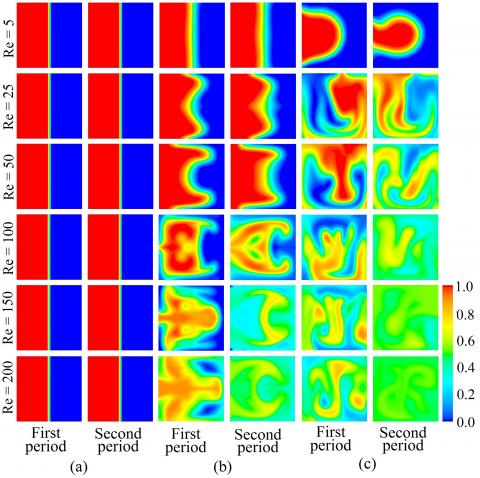Figure 6. Fluid2 volume fraction contours lines at the outlet of the first and second period of the three geometries for vertical injection: (a) Straight channel, (b) Serpentine-2D, (c) Chaotic geometry S-3D

In the S–2D geometry, the secondary flows are generated with more intensity than in the straight channel because of the existence of recirculation zones (Figure 7), where the inertial effects dominate. In this geometry, the increase of the mixing strongly depends on the injection mode. When the injection is horizontal (green line present the horizontal mode in Figure 7a), the two vortices still in the same region (fluid1 or fluid2) and the two fluids remain separated along the channel (Figure 5b). However, when the injection is vertical (red line present the vertical mode in Figure 7a), the vortices destroy the interface and, as a result, the mass transfer is enhanced. In addition, when the Reynolds number increases, mixing is more vigorous (Figure 6b). Due to the two vortices, the fluid at the middle of the S-2D geometry is forced toward the wall, while the fluid nearby the wall is forced toward the center. The strength of the vortices increases with increasing Reynolds number.

The vertical injection reveals that the flow is fully symmetrical in the type of this geometry (Figure 6b). This symmetry highly depends on the Reynolds number and it exists only in a flow where the regime is laminar. The destruction of this symmetry is an indication of the destabilization of the flow and it can be a precursor of the transitional regime onset. In the S–2D channel (Figure 8a), the flow is generally regular, and the particles trajectories do not diverge . Thereby, the S–2D geometry is not the appropriate geometry to achieve a perfect mixing.

In the S–3D channel, the particle trajectories can diverge rapidly. This property is a clear signature of chaotic advection (Figure 8b). Consequently, fluid particles can visit regions close to the walls. Thus, the stretching and refolding in the fluids lead the mixture to a fast and satisfactory uniformity. For a Reynolds number of 5, the inertial forces are negligible compared to the viscous forces which explain the weakness of the mixing. From a Reynolds number equal to 25, the mixing process in this geometry becomes notable due the secondary flows generated by the inertial effects (Figure 5c, and Figure 6c). Even if the injection is made according to one privileged transverse direction (either vertical or horizontal), the geometric perturbation in this geometry is three–dimensional. After sufficient distance, the effect of the privileged direction is almost completely cancelled.

It is necessary to note that each period of S-3D geometry influences the flow by the presence of its two perturbations: the first one makes the flow rotating in the positive direction (counter clockwise direction), while the second one generates a rotating flow in the negative direction (clockwise direction). Figure 7a–b show the secondary flows produced after the first and the second perturbation respectively. It is noticed that in figure 7a the positive vortice is dominant, while in figure 7b the negative vortice is dominant. Indeed, when the fluid is perturbed in the positive direction, the positive vortice dominates the flow, and when it is perturbed in the negative direction, the negative vortice will be dominant. This allows concluding that in the first perturbation, the positive vortice increases at the expense of the negative vortice, and in the second perturbation, the negative vortice increases at the expense of positive vortice. The consequence is the appearance of the stretching and refolding processes, which is a strong sign of chaotic advection. So, the serpentine–3D geometry reaches a rapid chaotic mixing in both injection modes compared to the two others geometries.

To quantify the mixing efficiency in the three geometries, the mixing degree is calculated according to Eq. (1) and as a function of Reynolds number. Figure 9 shows the evolution of the mixing degree with the Reynolds number for the three geometries for both injection modes (horizontal and vertical).

## 7.jpgFigure 7. Secondary flow at different cross section for the both geometries for Re = 100 ((a) middle of 1st period, (b) end of 1st period, (c) middle of 2nd period, and (d) outlet of 2nd period, green and red line are presents the interface between fluids in horizontal and vertical injection modes respectively)

## 8.jpg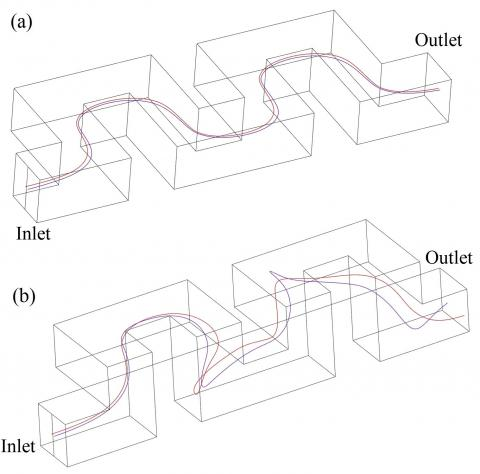Figure 8. Evolution of two particles trajectories after two periods, (a) Serpentine-2D, (b) Serpentine-3D

## 9.jpgFigure 9. Evolution of the mixing degree versus Reynolds number at the outlet of the three geometries. (a) Horizontal injection, (b) Vertical injection

The mixing degree is equal to zero for both injection modes regardless the Reynolds number in the straight channel, so it is unfavorable to efficient mixing, because under the laminar regime, the distance between particles stayed constant, and the interfacial area is stable without the molecular diffusion. In the S–2D geometry, when the interface between the two fluids is horizontal, the mixing did not occur at all and the mixing degree is equal, all the time, to zero (Figure. 9a). However, when the interface is vertical, the mixture appears and the mixing is more vigorous and the mixing degree evolves more quickly when the Reynolds number increases (Figure. 9b).

With initial horizontal and vertical injections, the mixing degree in S–3D geometry is almost identical and this geometry has the same mixing ability in both injection modes (Figure. 9). This is not the case for the S–2D geometry, where the flow rotations in the bends are increased the interfacial area in the vertical mode only. The mixing by chaotic advection is kinematic and accentuated by the Reynolds number augmentation. At Reynolds number equal to 200, the degree of mixing reaches the complete mixing, that may be defined when (D > 0.95). In the S–2D geometry, the Reynolds number equivalent to this asymptotic value (D > 0.95) is much greater than 200 or by increasing the period numbers.

Figure 10 presents, for two Reynolds numbers 100 and 200, the evolution of the mixing degree with the curvilinear coordinate for the three studied geometries and by considering the two injection modes. The mixing degree evolves increasingly with the curvilinear coordinate and the effectiveness of mixing is accentuated when the Reynolds number increases. In the straight channel, the mixing is due only to the diffusion effects at low Reynolds number meanwhile the molecular diffusion is not considered. So, the mixing degree is equal to zero. In the S–2D geometry, the right angles create extra agitation accentuating the mixing in the case of vertical injection. However, this agitation is not sufficient to have a rapid and a homogeneous mixing. The S–3D geometry produces chaotic regions in the flow and therefore provides high levels of mixing superior to 0.95 when Reynolds number equal to 200 .

3.3 Chaotic kinematic behavior of fluid particles

The quality of mixing is strongly correlated to both parameters: dispersion of particles and diverging trajectories. The techniques used in nonlinear dynamic systems are standard tools to examine the behavior of fluid particles in fluid mechanics such as Poincaré section and Lyapunov exponent, [14, 24, 25].

## 10.jpg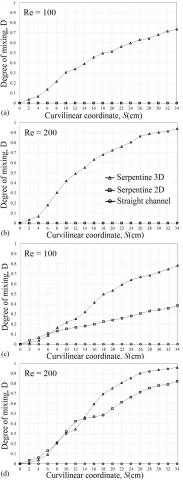Figure 10. Evolution of mixing degree as function of the curvilinear coordinate for different geometries, (a) and (b) horizontal injection, (c) and (d) vertical injection

These tools provide interesting information about the particle kinematics and they are based on the tracking of fluid particles. Especially, Poincaré section gives cartography on the flow structure by superimposing the intersections of several trajectories on a single plane. If the Poincaré sections present an organized curve, the flow is regular or periodic. If points of the Poincaré sections are disseminated or have a random distribution, the flow is chaotic.

Figure 11 shown the procedure used to generate the Poincaré section. In this study fourteen points of fluid particles initially located on the center line of the inlet section (see Figure 12a) (horizontally or vertically) of each geometry are released and tracked, and the intersection of these trajectories with planes (p1, p2, p8 in Figure 11a, and Figure 11b) is superimposed on a same plane (outlet section), (Figure 11c, and Figure 11d).

Figure 12 presents the Poincaré sections for two geometries (S–2D and S–3D) and for two Reynolds numbers 100 and 200. The particles are injected both horizontally and vertically. In the S–3D geometry (Figure 12b), and for the horizontal injection, the distribution of the particles is not homogenous and it presents a cloud of points. This explains that the particles cover all the cross section. The dissemination is accentuated with the increase of the Reynolds number. The injection mode in the S–3D geometry has no effect on the dispersion phenomena because the Poincaré sections are mostly unchanged. This behavior of the Poincaré sections demonstrates that the flow is chaotic and consequently led to the improvement of fluids mixing.

In the S–2D geometry (Figure 12c) and when the injection is horizontal the center line is barely affected by the two vortices created in the cross section which led to a very weak dispersion of the fluid particles for the two Reynolds numbers 100 and 200. In the vertical injection case, the Poincaré sections are more dispersed which results in better mixing. However, the flow in this geometry presents symmetrical streamlines between the superior and the inferior fluid regions. This symmetry depends strongly on the Reynolds number. In addition, the mixing between these regions (superior and inferior) does not occur which result in a less dispersion compared to that found in the S–3D geometry.

In Figure 13, the inlet section is divided into equal parts, horizontally and vertically. The fluid particles in the one region are marked by a red color while in the second region, the fluid particles are marked by the blue color. The particles are tracked in the two geometries and for the two Reynolds numbers (100, and 200). This figure outlines the evolution of the map structure with the curvilinear coordinate. In the S–2D geometry, the distribution shows that the flow is symmetrical. In others words, the upper region is completely identical to the lower one. Consequently, it is needed to inject vertically in order to mix well the fluids. It can be seen that the mixing is more vigorous when the Reynolds increases. However, in the S–3D geometry, due to the chaotic behavior, the map of fluid particles is unchanged regardless of the injection mode and the dispersion is accented with the augmentation of the Reynolds numbers.

To highlight the mechanism of mixing, we present the streamlines in the fluid flow for two values of Reynolds numbers (100 and 200) into all geometries, see Figure 14.

In the straight channel, the streamlines are parallels regardless the Reynolds number values, which explain that the mixing is very poor.

As for as the other geometries, different mechanisms take place in the flow such as twisting, vortices, splitting, and recombining.

## 11.jpgFigure 11. Trajectory of a particle passes through a set of plans in the geometries, (a) S-3D, and (b) S-2D to generate the Poincaré section, all intersections are plotted in a single plane (c) S-3D, and (d) S-2D

## 12.jpgFigure 12. Poincaré section in: (b) Serpentine-3D, and (c) Serpentine-2D, (a) Position of particles at inlet

## 13.jpgFigure 13. Fluids particles distribution in cross-section at: (a) middle of 1st period, (b) end of 1st period, (c) middle of 2nd period, and (d) outlet, for different injection modes and for Reynolds numbers 100 and 200

## 14.jpg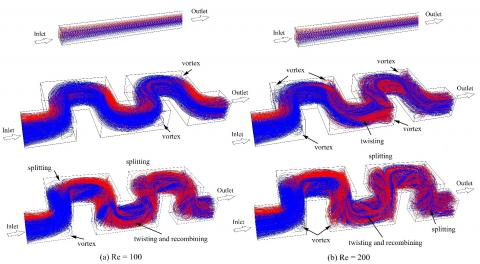Figure 14. Streamlines in the all geometries for Reynolds number: (a) Re = 100, and (b) Re = 200

The mixing occurs inside channels (S-2D and S-3D) after the splitting position due to the first geometrical perturbation in both geometries. The splits of fluid streams create vortices near the corners of channels, and generate transversal flows. Due to these vortices, fluids are temporarily imprisoned near the corners and facilitating the mixing between the both fluids. When the fluids exit the first geometrical perturbation, the fluid streams is recombined and twisted. We remark that these phenomena are very strong in the chaotic geometry compared to that showed in the S-2D geometry. These mechanisms allow, in one hand, the production of manifold very thin layers in S-3D channel and in other hand, they increase the interfacial contact and mass transfer between both fluids contrary to the S-2D geometry.

3.4 Heat transfer

3.4.1 The parietal heat transfer

In this study, the wall heat flux value is taken equal to 5000 W/m² and the inlet temperature is equal to 300 K. The evolution of the Nusselt number Nu along the curvilinear coordinate S was studied for two Reynolds number 100 and 200, for different geometries (Straight Channel, Serpentine-2D, and Serpentine-3D), see Figure 15.

In the straight channel, the evolution of the Nusselt number is decreasing and it is towards to an asymptotic value equal to 3.09. This value presents a good concordance with the literature . This result is a validation of the numerical method of our results. As mentioned above, the presence of vortices (see Figure 7) allows to the cold particles at the middle of the S-2D geometry to move toward the wall, while the hot particles nearby the wall is moved toward the center. This movement generates fluctuations of Nusselt number. After the entry zone where the Nusselt number decreases strongly, its evolution oscillates around a mean value which equal to 25.5 for Re=200, and 12.4 for Re=100. We can see that the mean Nusselt number increases with the increase of the Reynolds number.

In S-3D geometry, a periodic variation of the Nusselt number is observed after the entrance zone (S>0.02m) with a mean value of 39.97 and 25.76 for Reynolds number equal to 200 and 100 respectively. In this geometry, the heat transfer enhances due to the existence of chaotic advection regime and thus the mean value of the Nusselt number is greater than those obtained in the two other geometries.

## 15.jpgFigure 15. Evolution of the Nusselt number with the curvilinear coordinate for different geometries: (a) Re = 100, and (b) Re = 200

3.4.2 Thermal mixing (Temperature homogenization)

To compute the heat mixing rate between two fluids (hot and cold), a condition of adiabatic walls for each geometry is imposed. The inlet section is divided into two parts vertically. In the first part, the water is at a temperature of 300 K and in the other part is at 320 K.

## 16.jpgFigure 16. Evolution of thermal mixing degree as function of the curvilinear coordinate for different geometries, (a) Re = 100, and (b) Re = 200

## 17.jpgFigure 17. Evolution of the thermal mixing degree versus Reynolds number at the outlet of the three geometries

Figure 16 shows the evolution of thermal mixing degree with the curvilinear coordinate for the three studied geometries and by considering the vertical injection mode and for two Reynolds number 100 and 200. In the straight channel, the heat transfer is done by conduction which explains that the mixing degree evolves slowly comparing to the others geometries. In the two geometries (S-2D and S-3D), the thermal mixing increases more quickly with the curvilinear coordinate and the mixing efficiency is emphasized when the Reynolds number increases. In the S–2D geometry, the vortices create an agitation which accentuates the heat mixing however this agitation is not sufficient to have a good heat mixing rate. The S–3D geometry generates chaotic regions in the flow and therefore it provides mixing level value superior to 0.97 when the Reynolds number equal to 200 and 0.94 for Reynolds number equal to 100 at the outlet section. These values of the degree mixing are acceptable for a perfect quality of a thermal mixing.

Figure 17 presents the evolution of the thermal mixing degree as function of the Reynolds number at the outlet cross section of the three geometries. In the straight channel, the mixing degree decreases with the Reynolds number because the heat transfer is dominated by conduction mode. In the others geometries, the mixing degree evolves with the Reynolds number where its values are more important in the chaotic geometry.

4. Conclusion

The fluids mixing was numerically modeled by using CFD code in three geometries Straight channel, S–2D channel and S–3D channel. These geometries were examined in terms of particles dispersion, fluids mixing, thermal mixing and parietal heat exchange. In the straight channel, the flow is regular and the fluid particles trajectories are parallel. Consequently, there is no mixing of the two fluids.

The results show that serpentine–2D improves the mixing due to the existence of the recirculation zones but the mixing performances remain limited especially in the initial horizontal injection case where the mixing degree is equal, at all, to zero.

The volume fraction contours, degree of mixing, the Poincaré section, and the particles distribution confirm that the S–3D geometry mixes very well the two fluids and the height level quality reaches its asymptotic value for the S–3D geometry for both initial injection modes. This improvement is due to the stirring and folding promoted by chaotic advection. As for the heat transfer, the important Nusselt number is obtained for the S-3D geometry even for the mixing rate where the perfect quality is computed in the same geometry. This study showed clearly that the S–3D geometry can offer a number of advantages in the engineering applications as a promoted mixing process. An experimental is in progress to evaluate the performance of the chaotic geometry as mixer.

References

 Kaci H.M., Lemenand T., Della V.D., Peerhossaini H. (2009). Effects of embedded streamwise vorticity on turbulent mixing, Chemical Engineering and Processing, Vol. 48, pp. 1457-1474. DOI: 10.1016/J.CEP.2009.08.002

 Aref H. (1984). Stirring by chaotic advection, J Fluid Mech, Vol. 143, pp. 1-21.

 Raynal F., Gence J. (1997). Energy saving in chaotic laminar mixing, International Journal of Heat and Mass Transfer, Vol. 40, No. 14, pp. 3267-3273. DOI: 10.1016/S0017-9310(96)00383-3

 Jones S.W., Thomas O.M., Aref H. (1989). Chaotic advection by laminar flow in twisted pipe, J Fluid Mech, Vol. 209, pp. 335-357. DOI: 10.1017/S0022112089003137

 Acharya N., Sen M., Chang H.C. (1992). Heat transfer enhancement in coiled tubes by chaotic advection, International Journal of Heat and Mass Transfer, Vol. 35, pp. 2475-2489.

 Peerhossaini H., Castelain C., Le Guer Y. (1993). Heat exchanger design based on chaotic advection, Exp Therm Fluid Sci, Vol. 7, pp. 333-344. DOI: 10.1016/0894-1777(93)90056-O

 Castelain C., Mokrani A., Le Gentil Homme P., Peerhossaini H. (1997). Residence time distribution in twisted pipe flows: helically coiled system and chaotic system, Exp Fluids, Vol. 22, pp. 359-368. DOI: 10.1007/S003480050061

 Lemenand T., Peerhossaini H. (2002). A thermal model for prediction of the nusselt number in a pipe with chaotic flow, Appl Therm Eng, Vol. 22, pp. 1717-1730. DOI: 10.1016/S1359-4311(02)00075-3

 Habchi C., Lemenand T., Peerhossaini H. (2009). Liquid–liquid dispersion in a chaotic advection flow, Int J Multiphase Flow, Vol. 35, pp. 485-497. DOI: 10.1016/J.IJMULTIPHASEFLOW.2009.02.019

 Zhou T.G., Xu Y., Liu Z., Joo S.W. (2015). An enhanced one-layer passive microfluidic mixer with an optimized lateral structure with the dean effect, ASME J Fluid Eng, Vol. 137, No. 9, pp. 091102. DOI: 10.1115/1.4030288

 Kang T.G., Hulsen M.A., Anderson P.D. (2007). Chaotic advection using passive and externally actuated particles in a serpentine channel flow, Chemical Engineering Science, Vol. 62, pp. 6677-6686. DOI: 10.1016/J.CES.2007.07.044

 Lin K.W., Yang J.T. (2007). Chaotic mixing of fluids in a planar serpentine channel, International Journal of Heat and Mass Transfer, Vol. 50, pp. 1269-1277. DOI: 10.1016/J.IJHEATMASSTRANSFER.2006.09.016

 Habchi C., Harison J.L., Russeil S., Bougeard D. (2013). Chaotic mixing by longitudinal vorticity, Chemical Engineering Science, Vol. 104, pp. 439-450. DOI: 10.1016/J.CES.2013.09.032

 Karami M., Shirani E., Jarrahi M., Peerhossaini H. (2014). Mixing by time-dependent orbits in spatiotemporal chaotic advection, ASME J Fluid Eng, Vol. 137, No. 1, pp. 011201. DOI: 10.1115/1.4027588

 Robin H.L., Stremler A.M., Sharp K.V., Olsen M.G., Santiago J.G., Aref H., Beebe D.J. (2000). Passive mixing in a three-dimensional serpentine microchannel, Journal of Micro electro mechanical Systems, Vol. 9, No. 2, pp. 190-197. DOI: 10.1109/84.846699

 Hwang F.N., Cai X.C., Cheng Y.L. (2013). A parallel fully coupled implicit domain decomposition method for numerical simulation of microfluidic mixing in 3D, International Journal of Computer Mathematics, Vol. 90, No. 3, pp. 615-629. DOI: 10.1080/00207160.2012.727988

 Lasbet Y., Auvity B., Castelain C., Peerhossani H. (2006). A chaotic heat-exchanger for PEMFC cooling applications, Journal of Power Sources, Vol. 156, pp. 114-118. DOI: 10.1016/J.JPOWSOUR.2005.08.030

 Lasbet Y., Auvity B., Castelain C., Peerhossani H. (2007). Thermal and hydrodynamic performances of chaotic mini-channel: application to the fuel cell cooling, Heat Transfer Engineering, Vol. 28, No. 8–9, pp. 795-803. DOI: 10.1080/01457630701328908

 Castelain C., Lasbet Y., Auvity B., Peerhossani H. (2016). Experimental study of the thermal performance of chaotic geometries for their use in PEM Fuel Cells, International Journal of Thermal Sciences, Vol. 101, pp. 181-192.

 Beebe D., Adrian R., Olsen M., Stremler M., Aref H., Jo B. (2001). Passive mixing in micro–channels: fabrication and flow experiments, Mécanique et Industries, Vol. 2, pp. 334-348.

 Liu Y.Z., Kim B.J., Sung H.J. (2004). Two–fluid mixing in a microchannel, Int J Heat and Fluid Flow, Vol. 25, pp. 986-995.

 Cook K.J., Fan Y.F., Hassan I. (2013). Mixing evaluation of a passive scaled-up serpentine micromixer with slanted grooves, ASME J Fluid Eng, Vol. 135, No. 8, pp. 081102. DOI: 10.1115/1.4024146

 Hongjun S., Ziliang C., Hongseok N., Dawn J.B. (2010). Chaotic mixing in microchannels via low frequency switching transverse electroosmotic flow generated on integrated microelectrodes, The Royal Society of Chemistry Lab Chip, Vol. 10, pp. 734-740. DOI: 10.1039/B918213F

 Ottino J.M. (1989). The kinematics of mixing: stretching, chaos and transport, Cambridge University Press, Cambridge, UK.

 Toussaint V., Carrière P., Raynal F. (1995). A numerical eulerian approach to mixing by chaotic advection, Physics of Fluids, Vol. 7, No. 11, pp. 2587-2600. DOI: 10.1063/1.868707

 Shah R.K., London A.L. (1978). Laminar flow forced convection in ducts, Academic Press, New York.